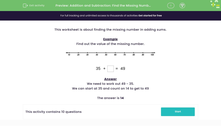# Find the Difference Using Addition or Subtraction

In this worksheet, students will use addition or subtraction to find the missing number.Key stage:  KS 1

Curriculum topic:   Number: Addition and Subtraction

Curriculum subtopic:   Understand Relationship Between Addition and Subtraction

Difficulty level:#### Worksheet Overview

Example

Find the value of the missing number.35 + = 49

We need to find the difference between the numbers 35 and 49.

We can start at 35 and count on to get to 49. This would be 14 numbers:  35 + 14 = 49

We could also start at 49 and count back to 35.  This would also be 14 numbers: 49 - 35 = 14

Both methods are correct and give us the same answer of 14. Which method works best for you?### What is EdPlace?

We're your National Curriculum aligned online education content provider helping each child succeed in English, maths and science from year 1 to GCSE. With an EdPlace account you’ll be able to track and measure progress, helping each child achieve their best. We build confidence and attainment by personalising each child’s learning at a level that suits them.

Get started